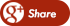﻿ Heat vs. Current in a ResistorBack

## Heat vs. Current in a Resistor# Heat vs. Current in a Resistor

## Energy can never be destroyed

It is converted from one form to another. One of the most familiar forms of energy is heat.

When a current (I) is forced through a resistor (R) by applying a potential (V), the electrical energy is converted to heat energy as observed by the rise in temperature of the resistor. Remember that power is the rate at which energy is consumed.

The energy dissipated in the resistor then is equivalent to the power it consumes multiplied by the length of time current is flowing. In this case, electrical power (in units of Watts) consumed by a resistor is equivalent to the product of applied Voltage and the Current flowing through it. This is called active or real power.

Note: Heat is only produced by a resistive load (electrical friction) and not in an inductive or capacitive load.

The value of the real power (P) consumed by the resistor is:

Power = Voltage x Current (P = V x I)

Since we already know that an applied Voltage (V) is required to force Current (I) through aresistor (R), another way of defining Power is:

Power = (Current)2 x Resistance (P = I2 x R)

Note: Real power only occurs when the magnitude of the voltage and current increase and decrease at exactly the same rate as illustrated below.

This is called being in-phase and will only happen for a resistive load.